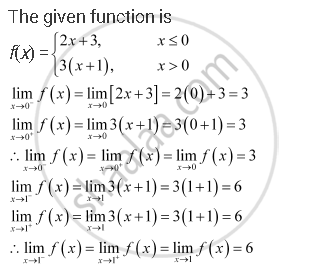CBSE (Arts) Class 11CBSE
Share

# Find Lim_(X -> 0) F(X) and Lim_(X -> 1) F(X) Where F(X) = {(2x + 3, X <= 0),(3(X+1), X > 0):} - CBSE (Arts) Class 11 - Mathematics

ConceptLimits Limits of Polynomials and Rational Functions

#### Question

Find  lim_(x -> 0) f(x) and lim_(x -> 1) f(x) where f(x) = {(2x + 3, x <= 0),(3(x+1), x > 0):}

#### SolutionIs there an error in this question or solution?

#### APPEARS IN

NCERT Solution for Mathematics Textbook for Class 11 (2018 to Current)
Chapter 13: Limits and Derivatives
Q: 23 | Page no. 302

#### Video TutorialsVIEW ALL 

Solution Find Lim_(X -> 0) F(X) and Lim_(X -> 1) F(X) Where F(X) = {(2x + 3, X <= 0),(3(X+1), X > 0):} Concept: Limits - Limits of Polynomials and Rational Functions.
S